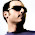## Monday, September 1, 2008

### How to Post Math Equations in Blogger using ASCIIMathMIL

I know that most of us working directly with mathematics are looking for a fundamental solution to posting equations on blogger. So far, I would have my equations written in MathType, print the screen, paste, crop, and save in paint. It is a pain... a dual monitor setup would be helpful, nonethless, this is too much to go through.

I've seen several models over the net that provide different solutions, but none of them was what I was looking for, especially that I am not a LaTeX guru.

So far, the neatest model to post equations on blogger is described on Fundamental Thinking. The method uses Peter Jepsen's ASCIIMathML script.

Here's how to set it up on your blog:

1. Download ASCIIMathML.js and host it somewhere online such as your google pages website. This will allow you to modify the script to fit your needs (like changing the forecolor of the equations). Alternatively, you can just point to the location of the script (http://www1.chapman.edu/~jipsen/mathml/ASCIIMathML.js)
2. In Blogger, add a new HTML/Java Script widget
3. Add the following to the new widget
<script src="http://www1.chapman.edu/~jipsen/mathml/ASCIIMathML.js" type="text/javascript"></script>
(In case you decided to host the script on your own website, you will need to replace src with the correct location).
Voila!

Now you're all set. All you need to to do is either learn the ASCIIMathML syntax, type in LaTeX, or copy from Mathtype as LaTeX.

To type in LaTeX, you have to wrap your equations with a dollar sign.

Alternatively, you can use some online LaTeX editors such as Thornahawk's Numerical Analysis notes or CodeCogs', and then copy and paste the code into your post.

Finally, if you use MathType, you can set it up so that it copies the equations as LaTeX. Here's how you do it
1. In Mathtype, go to
Preferences/Translators
2. Select "Translation to other language"
3. Select "TeX -- LaTeX 2.09 and later"
4. Deselect "include MathType data in translation"
Make sure you replace the $and$ generated by MathType by the dollar sign.

This, I think, is by far the easiest way of getting your equations into blogger. Sometimes, the translation generated by MathType is not well interpreted by the ASCIIMathML script. For example, type the following in MathType

$\lim_{x\rightarrow 0}$

This will not be translated correctly with the ASCIIMathML.

After all this, I think we need a better solution.

UPDATE: As Bob Mathews from design science (the makers of MathType) has kindly noted, if you choose the Format/Inline Equation in MathType, the LaTeX code will exclude the $and$ symbols.

Cite as:
Saad, T. "How to Post Math Equations in Blogger using ASCIIMathMIL". Weblog entry from Please Make A Note. https://pleasemakeanote.blogspot.com/2008/09/how-to-post-math-equations-in-blogger.html

1.Thanks -- good suggestions. I have one question and two comments.

Question: In your list of "how to set it up on your blog", your step #3 says to "add the following to the new widget", but shouldn't there be something following?

Comment #1: In your list of steps to create the LaTeX with MathType, if you'll also add the step "In the Format menu, choose the Inline Equation command", your statement about replacing the $and$ becomes unnecessary.

Comment #2: Your assessment of the problems in getting some LaTeX into ASCIIMathML is correct in that ASCIIMathML, while a great product, doesn't recognize all LaTeX commands, \mathop and \limits being two of the commands it does not recognize.

Bob Mathews
Design Science

2.Thank you for your feedback.

I added the link to the script (it was lost within the html).

Also, thanks for the note about enabling the inline equation option.

3.awesome!
This works great... LaTeX everywhere in my life.

4.Hey its great trick to use ASCIIMathMIL for making maths equation and I think in this there will be no need of cross product calculator or other calculator.